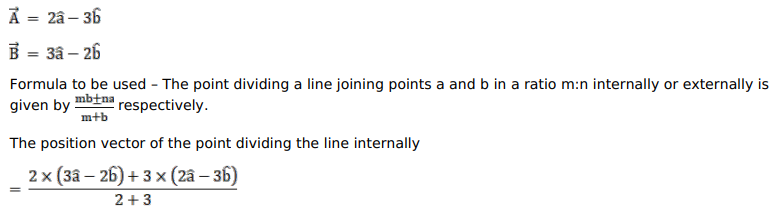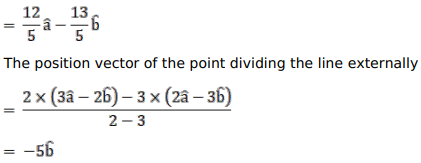# Find the position vector of the point which divides the join of the points

Question:

Find the position vector of the point which divides the join of the points $(2 \vec{a}-3 \vec{b})$ and $(3 \vec{a}-2 \vec{b})$ (i) internally and (ii) externally in the ratio $2: 3$.

Solution: